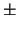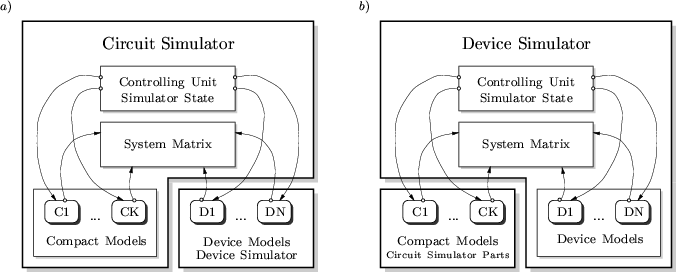Next: 5. Contacts and Boundaries Up: 4. Simulator Coupling Previous: 4.2 The Quasi Full-Newton

# 4.3 The Full-Newton Algorithm

The approaches discussed above combined a circuit simulator with a device simulator by adding a suitable interface to the circuit simulator. These approaches are easy to implement as only marginal changes in both simulators are required. The circuit simulator acts as server which controls the device simulator. At each Newton iteration of the circuit, an input deck for the device simulator has to be generated and the device simulator has to be called to calculate currents and conductances.

The approach taken in MINIMOS-NT is completely different. The capability to solve circuit equations was added to the simulator kernel. This allowed for assembling the circuit and the device equations into one system matrix which results in a real full-Newton method. There is no need to explicitly calculate the derivatives of the contact currents with respect to the contact voltages as the contact currents are solution variables which simply gives1 as a derivative in the constitutive relations.

However, the benefits gained from using the numerous existing SPICE models must not be neglected. As SPICE has a well defined and documented interface, it is, in principle, straight-forward to implement a similar interface in MINIMOS-NT. SPICE was originally written in FORTRAN and has been ported to C (SPICE3). Extensive use had been made of C-preprocessor macros which caused some software-technical difficulties when designing a neat C++ interface as needed by MINIMOS-NT. Mapping the internal state of MINIMOS-NT for the SPICE models was also quite tedious. However, these problems have been nicely solved in  where a tool is presented which automatically generates C++ source code for MINIMOS-NT. These source files can be compiled and either directly linked to MINIMOS-NT or dynamically loaded at runtime. The second approach allows for a compact simulator which can be extended whenever needed.A comparison of these different architectures is shown in Fig. 4.3. In Fig. 4.3a the device simulator acts as a client to the circuit simulator whereas in Fig. 4.3b the device simulator is extended with circuit simulator capabilities and can reuse circuit simulator models on demand.Next: 5. Contacts and Boundaries Up: 4. Simulator Coupling Previous: 4.2 The Quasi Full-Newton
Tibor Grasser
1999-05-31